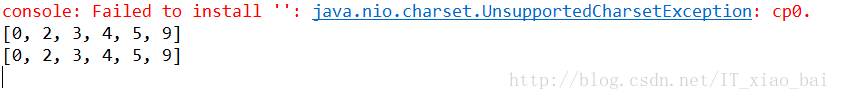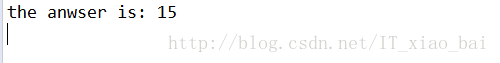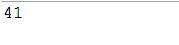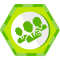## Java调用Python程序方法总结(最全最详细) 转

1. 在java类中直接执行python语句

• Jython是一种完整的语言，而不是一个Java翻译器或仅仅是一个Python编译器，它是一个Python语言在Java中的完全实现。Jython也有很多从CPython中继承的模块库。最有趣的事情是Jython不像CPython或其他任何高级语言，它提供了对其实现语言的一切存取。所以Jython不仅给你提供了Python的库，同时也提供了所有的Java类。这使其有一个巨大的资源库。

` `
1. `<dependency>`

2. `<groupId>org.python</groupId>`

3. `<artifactId>jython-standalone</artifactId>`

4. `<version>2.7.0</version>`

5. `</dependency>`

` `
1. `PythonInterpreter interpreter = new PythonInterpreter();`

2. `interpreter.exec("a=[5,2,3,9,4,0]; ");`

3. `interpreter.exec("print(sorted(a));"); //此处python语句是3.x版本的语法`

4. `interpreter.exec("print sorted(a);"); //此处是python语句是2.x版本的语法`-Dpython.console.encoding=UTF-82. 在java中调用本地python脚本

` `
1. `def add(a,b):`

2. `return a + b`

python的功能函数已经写好，接下来我们写一个java的测试类（同样需要用到Jpython包），来测试一下是否可以运行成功。代码如下：

` `
1. `import org.python.core.PyFunction;`

2. `import org.python.core.PyInteger;`

3. `import org.python.core.PyObject;`

4. `import org.python.util.PythonInterpreter;`

5.
6. `public class Java_Python_test {`

7.
8. `public static void main(String[] args) {`

9. `// TODO Auto-generated method stub`

10. `PythonInterpreter interpreter = new PythonInterpreter();`

11. `interpreter.execfile("D:\\add.py");`

12.
13. `// 第一个参数为期望获得的函数（变量）的名字，第二个参数为期望返回的对象类型`

14. `PyFunction pyFunction = interpreter.get("add", PyFunction.class);`

15. `int a = 5, b = 10;`

16. `//调用函数，如果函数需要参数，在Java中必须先将参数转化为对应的“Python类型”`

17. `PyObject pyobj = pyFunction.__call__(new PyInteger(a), new PyInteger(b)); `

18. `System.out.println("the anwser is: " + pyobj);`

19. `}`

20. `}`OK，测试成功了~

### 3. 使用Runtime.getRuntime()执行脚本文件（推荐）

` `
1. `import numpy as np`

2.
3. `a = np.arange(12).reshape(3,4)`

4. `print(a)`

` `
1. `import java.io.BufferedReader;`

2. `import java.io.IOException;`

3. `import java.io.InputStreamReader;`

4.
5. `public class Demo1 {`

6.
7. `public static void main(String[] args) {`

8. `// TODO Auto-generated method stub`

9. `Process proc;`

10. `try {`

11. `proc = Runtime.getRuntime().exec("python D:\\demo1.py");// 执行py文件`

12. `//用输入输出流来截取结果`

13. `BufferedReader in = new BufferedReader(new InputStreamReader(proc.getInputStream()));`

14. `String line = null;`

15. `while ((line = in.readLine()) != null) {`

16. `System.out.println(line);`

17. `}`

18. `in.close();`

19. `proc.waitFor();`

20. `} catch (IOException e) {`

21. `e.printStackTrace();`

22. `} catch (InterruptedException e) {`

23. `e.printStackTrace();`

24. `} `

25. `}`

26. `}`` `
1. `import sys`

2.
3. `def func(a,b):`

4. `return (a+b)`

5.
6. `if __name__ == '__main__':`

7. `a = []`

8. `for i in range(1, len(sys.argv)):`

9. `a.append((int(sys.argv[i])))`

10.
11. `print(func(a,a))`

` `
1. `int a = 18;`

2. `int b = 23;`

3. `try {`

4. `String[] args = new String[] { "python", "D:\\demo2.py", String.valueOf(a), String.valueOf(b) };`

5. `Process proc = Runtime.getRuntime().exec(args1);// 执行py文件`

6.
7. `BufferedReader in = new BufferedReader(new InputStreamReader(proc.getInputStream()));`

8. `String line = null;`

9. `while ((line = in.readLine()) != null) {`

10. `System.out.println(line);`

11. `}`

12. `in.close();`

13. `proc.waitFor();`

14. `} catch (IOException e) {`

15. `e.printStackTrace();`

16. `} catch (InterruptedException e) {`

17. `e.printStackTrace();`

18. `}`OK，成功了。

bat文件：

` `
1. `@echo off `

2. `C:`

3. `cd C:\Anaconda2\envs\py3`

4. `start python test.py`

5. `exit`

java程序：

` `
1. `import java.io.BufferedReader;`

2. `import java.io.IOException;`

3. `import java.io.InputStreamReader;`

4.
5. `public class Demo1 {`

6.
7. `public static void main(String[] args) {`

8. `// TODO Auto-generated method stub`

9. `Process proc;`

10. `try {`

11. `proc = Runtime.getRuntime().exec("cmd /c E:\\Faultdetection\\fault1\\run.bat");// 执行py文件`

12. `//用输入输出流来截取结果`

13. `BufferedReader in = new BufferedReader(new InputStreamReader(proc.getInputStream()));`

14. `String line = null;`

15. `while ((line = in.readLine()) != null) {`

16. `System.out.println(line);`

17. `}`

18. `in.close();`

19. `proc.waitFor();`

20. `} catch (IOException e) {`

21. `e.printStackTrace();`

22. `} catch (InterruptedException e) {`

23. `e.printStackTrace();`

24. `}`

25. `}`

26. `}`### 短短的歼击机Anoyi
2018/09/29
0
0
（转载）Python 的 JPype 模块调用 Jar 包

Python 的 JPype 模块调用 Jar 包 import os.path startJVM("F:/Java/jdk1.8.0_45/jre/bin/server/jvm.dll", "-ea") java.lang.System.out.println("hello World") shutdownJVM() import jp......

2018/03/21
0
0
Python or Java？Boss直聘告诉你该如何选择

CSDN程序人生
2018/09/11
0
0
Java中如何调用python的API？

2012/05/08
4.1K
3
Py4J —— Python 和 Java 的互调接口

Py4J 使得 Python 程序可以利用 Python 解释器直接调用Java虚拟机中的 Java 对象，也可以让 Java 调用 Python 对象，有点像 Python 版的 JNI。...

2010/10/02
264
0

7
0
javascript-十六进制随机颜色

<script> // 编写一个函数，获得一个十六进制的随机颜色的字符串（如#20CD4F） // function randomColor(){ // var r = random(0,255).toString(16); // var g = random(0,255).toString(16......

ACKo

3
0
springBoot +mybatis 出现sql 语句在数据库可以查询到，但是赋值到实体类上就没有的情况？

1.不要老是反复查看自己是否写错了，为啥有的能出来有的出不来？ 可以查看配置文件中是否配置全： 如果在application.yml 文件中是如下配置： mybatis: mapper-locations: classpath:mapp...

kuchawyz

3
0

wytao1995

3
0

2
0